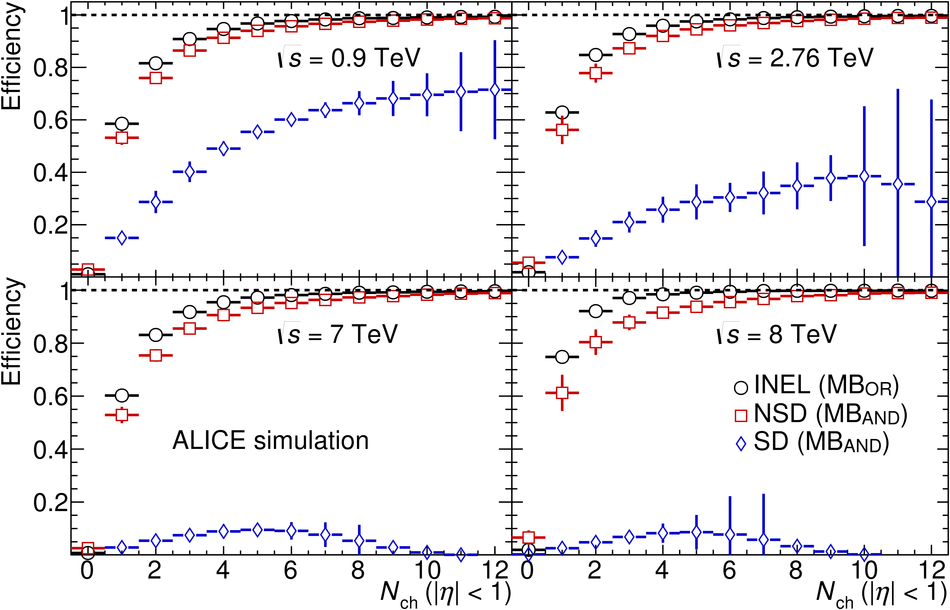# Figure 2

 Charged-particle multiplicity ($\nch =$ number of primary charged particles generated in $|\eta| < 1$) dependence of the efficiency of the event selection described in Section 4, obtained as the average between PYTHIA6 Perugia0 and PHOJET, both tuned for single diffraction defined for $M_X < 200$ GeV/$c^2$ (see ). Efficiencies are given for INEL events with MB$_{\rm{OR}}$ trigger (open circles), NSD events with \mband trigger (open squares), and SD events with MB$_{\rm{AND}}$ trigger (open diamonds), at $\sqrt{s} =$ 0.9 TeV (top left), 2.76 TeV (top right), 7 TeV (bottom left) and 8 TeV (bottom right). Error bars correspond to the difference between the two event generators and statistical uncertainty added in quadrature (non-negligible only for the SD events selection efficiency).